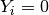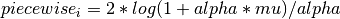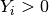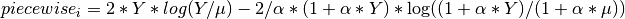# statsmodels.genmod.families.family.NegativeBinomial.resid_dev¶

NegativeBinomial.resid_dev(endog, mu, scale=1.0)[source]

Negative Binomial Deviance Residual

Parameters: endog : array-like endog is the response variable mu : array-like mu is the fitted value of the model scale : float, optional An optional argument to divide the residuals by scale resid_dev : array The array of deviance residuals

Notes

resid_dev = sign(endog-mu) * sqrt(piecewise)

where piecewise is defined as

If:If: